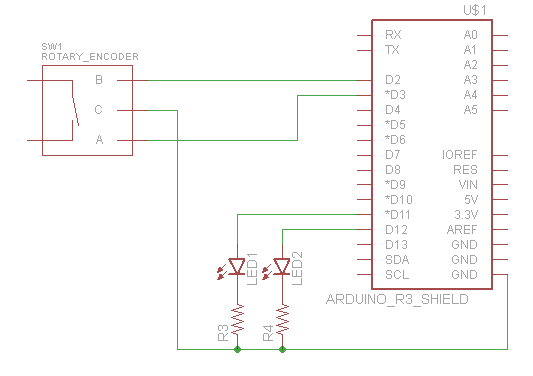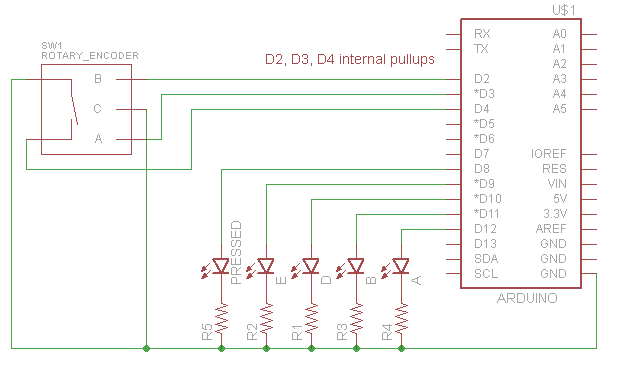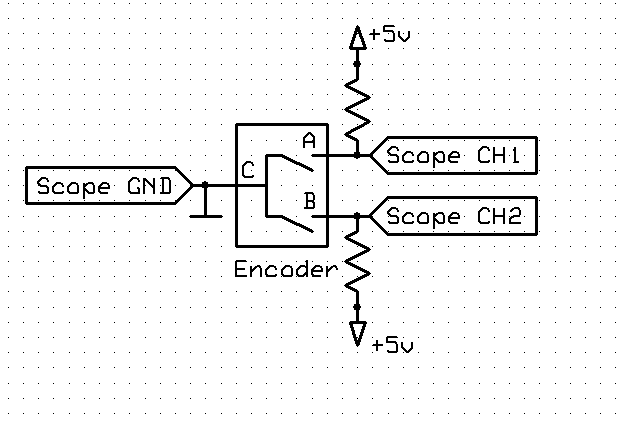# Using a rotary encoder: simple tutorial

Hi all,

Thought I'd share this code, which might help de-mystify the inards of a rotary encoder. It's not intended as a real-life solution- there are libraries for that and interrupts seems to be the way to go. It's more of a tutorial so you can see how the 2 outputs vary as the encoder is rotated.

Attached is:

• A snippet from the EC11 datasheet. It shows there are 2 detents (detent = click stop) per cycle but mine only has one.
• My schematic
• Sketch encoder_v3 which just uses 2x LEDs to indicate the pins' status, and it also prints an A, a, B and b depending on that status. You have to turn very slowly between detents to see these.
• Sketch encoder_v4 which counts the cycles, taking account of direction, and prints where you are.

Use it, don't use it 8). As always, YMMV.Jim

encoder_v3.ino (1.75 KB)

encoder_v4.ino (3.57 KB)

Mark2 of my encoder sketch is attached.

My encoder has a push-to-make switch in the knob, so I used that to double up and give 2 independent encoders. Seeing as the pins on the encoder are A and B, I re-used them as D and E; C is the middle pin.

If you run it with the serial monitor open, you'll see it keeps track of where you are with the knob either pressed or not.encoder_v5.ino (6.1 KB)

Added scope outputs from turning in either direction.

(I just bought a scope 8))

Edit... by the way, no Arduino involved there, just pulled each channel high with a 20k to 5V; see attached pic

Test circuit:One click clockwise:

one click clockwise.bmp (219 KB)

One click anticlockwise:

one click anti clockwise.bmp (219 KB)

recently a german user posted this code that takes care of all the special situations a mechanical-switch-encoder produces if you change moving direction.

Standard-encoder code fails to count right if the direction-reversion occurs if the mechanical contacts are not exactly
"in the middle" of the contact-zone.

``````const byte ENCODER_A_PIN = 3;
const byte ENCODER_B_PIN = 2;
const byte SWITCH_PIN = 4;

void setup() {
Serial.begin(115200);
Serial.println("\nStart");
pinMode(ENCODER_A_PIN, INPUT);
pinMode(ENCODER_B_PIN, INPUT);
pinMode(SWITCH_PIN, INPUT);
}

void loop() {
int8_t state = 0;
if (rotaryEncoder(state)) {
Serial.println("- SWITCH -");
}
if (state == -1) {
Serial.println("<-- ");
}
if (state == 1) {
Serial.println(" -->");
}
}

bool rotaryEncoder(int8_t &delta) {
delta = 0;
enum {STATE_LOCKED, STATE_TURN_RIGHT_START, STATE_TURN_RIGHT_MIDDLE, STATE_TURN_RIGHT_END, STATE_TURN_LEFT_START, STATE_TURN_LEFT_MIDDLE, STATE_TURN_LEFT_END, STATE_UNDECIDED};
static uint8_t encoderState = STATE_LOCKED;
static bool switchState = s;
switch (encoderState) {
case STATE_LOCKED:
if (a && b) {
encoderState = STATE_UNDECIDED;
}
else if (!a && b) {
encoderState = STATE_TURN_LEFT_START;
}
else if (a && !b) {
encoderState = STATE_TURN_RIGHT_START;
}
else {
encoderState = STATE_LOCKED;
};
break;
case STATE_TURN_RIGHT_START:
if (a && b) {
encoderState = STATE_TURN_RIGHT_MIDDLE;
}
else if (!a && b) {
encoderState = STATE_TURN_RIGHT_END;
}
else if (a && !b) {
encoderState = STATE_TURN_RIGHT_START;
}
else {
encoderState = STATE_LOCKED;
};
break;
case STATE_TURN_RIGHT_MIDDLE:
case STATE_TURN_RIGHT_END:
if (a && b) {
encoderState = STATE_TURN_RIGHT_MIDDLE;
}
else if (!a && b) {
encoderState = STATE_TURN_RIGHT_END;
}
else if (a && !b) {
encoderState = STATE_TURN_RIGHT_START;
}
else {
encoderState = STATE_LOCKED;
delta = -1;
};
break;
case STATE_TURN_LEFT_START:
if (a && b) {
encoderState = STATE_TURN_LEFT_MIDDLE;
}
else if (!a && b) {
encoderState = STATE_TURN_LEFT_START;
}
else if (a && !b) {
encoderState = STATE_TURN_LEFT_END;
}
else {
encoderState = STATE_LOCKED;
};
break;
case STATE_TURN_LEFT_MIDDLE:
case STATE_TURN_LEFT_END:
if (a && b) {
encoderState = STATE_TURN_LEFT_MIDDLE;
}
else if (!a && b) {
encoderState = STATE_TURN_LEFT_START;
}
else if (a && !b) {
encoderState = STATE_TURN_LEFT_END;
}
else {
encoderState = STATE_LOCKED;
delta = 1;
};
break;
case STATE_UNDECIDED:
if (a && b) {
encoderState = STATE_UNDECIDED;
}
else if (!a && b) {
encoderState = STATE_TURN_RIGHT_END;
}
else if (a && !b) {
encoderState = STATE_TURN_LEFT_END;
}
else {
encoderState = STATE_LOCKED;
};
break;
}

uint32_t current_time = millis();
static uint32_t switch_time = 0;
const uint32_t bounce_time = 30;
bool back = false;
if (current_time - switch_time >= bounce_time) {
if (switchState != s) {
switch_time = current_time;
back = s;
switchState = s;
}
}
return back;
}
``````

best regards Stefan

The german users code work really well for me on my ESP32.

I also found this blog post very useful.
Rotary Encoder : How to use the Keys KY-040 Encoder on the Arduino

Specifically the very last code example

``````// Robust Rotary encoder reading
//
// Copyright John Main - best-microcontroller-projects.com
//
#define CLK 2
#define DATA 7

void setup() {
pinMode(CLK, INPUT);
pinMode(CLK, INPUT_PULLUP);
pinMode(DATA, INPUT);
pinMode(DATA, INPUT_PULLUP);
Serial.begin (115200);
Serial.println("KY-040 Start:");
}

static uint8_t prevNextCode = 0;
static uint16_t store=0;

void loop() {
static int8_t c,val;

c +=val;
Serial.print(c);Serial.print(" ");

if ( prevNextCode==0x0b) {
Serial.print("eleven ");
Serial.println(store,HEX);
}

if ( prevNextCode==0x07) {
Serial.print("seven ");
Serial.println(store,HEX);
}
}
}

// A vald CW or  CCW move returns 1, invalid returns 0.
static int8_t rot_enc_table[] = {0,1,1,0,1,0,0,1,1,0,0,1,0,1,1,0};

prevNextCode <<= 2;
prevNextCode &= 0x0f;

// If valid then store as 16 bit data.
if  (rot_enc_table[prevNextCode] ) {
store <<= 4;
store |= prevNextCode;
//if (store==0xd42b) return 1;
//if (store==0xe817) return -1;
if ((store&0xff)==0x2b) return -1;
if ((store&0xff)==0x17) return 1;
}
return 0;
}
``````